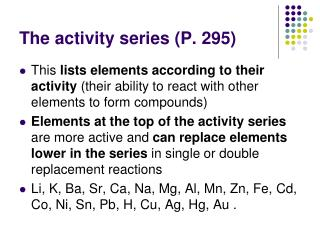DownloadDownload PresentationThe activity series (P. 295)

# The activity series (P. 295)

Télécharger la présentation## The activity series (P. 295)

- - - - - - - - - - - - - - - - - - - - - - - - - - - E N D - - - - - - - - - - - - - - - - - - - - - - - - - - -
##### Presentation Transcript

1. The activity series (P. 295) • This lists elements according to their activity (their ability to react with other elements to form compounds) • Elements at the top of the activity series are more active and can replace elements lower in the series in single or double replacement reactions • Li, K, Ba, Sr, Ca, Na, Mg, Al, Mn, Zn, Fe, Cd, Co, Ni, Sn, Pb, H, Cu, Ag, Hg, Au .

2. Activity Series (contd.) • For the following reactions, predict if they are possible and balance them….. • 1) Al+Fe(NO3)3 Fe+Al(NO3)3 • 2) Li+H2O LiOH+H2 • 3) FeSO4+ Cu CuSO4+ Fe • 4) Al2O3 + Fe  Fe2O3 + Al

3. Chapter 10 – The Mole • An atom has a tiny mass (≈ 10-23 g ), we must therefore use an appropriately small unit…. The atomic mass unit or amu • Carbon -12 has a mass of exactly 12 amu • To find the formula mass of any molecule or unit of a compound, find the # of atoms of each element, multiply this # by atomic mass of the element and finally, add up all of the elements present.

4. Atomic mass and Formula Mass • Example: Find the formula mass of H2SO4. 2 X Hydrogen = 2 X1 = 2amu 1 X Sulfur = 1 X32 = 32amu 4 X Oxygen = 4 X 16 = 64amu Total Formula mass 98 amu

5. Find the formula mass each of the following substances….. • 1) Al+Fe(NO3)3 Fe+Al(NO3)3 • 2) Li+H2O LiOH+H2 • 3) FeSO4+ Cu CuSO4+ Fe • 4) Al2O3 + Fe  Fe2O3 + Al

6. Moles • The number of atoms needed to make up an element’s atomic number in grams. • Example: 15.99g of Oxygen 63.55g of Copper 12.011g of Carbon 1.00g of Hydrogen All are composed of 6.02 X 1023 atoms The number is also known as Avogadro’s number

7. Moles and Molar Mass • For molecules (substances formed by atoms joined by covalent bonds) a mole of a compound is 6.02 X 1023 molecules of the compound. • Example: 1 mole of water has a mass of 2 X 1g = 2g + 1 X 16g = 16g 1mole of water = 18g and has 6.02 X 1023 molecules 18g/mol is known as the Molar Mass of a compound

8. How big is Avogadro’s number? • 6.02 X 1023 sheets of notebook paper stacked would go beyond our solar system. • 6.02 X 1023 footballs would form another planet bigger than the earth. • 6.02 X 1023 popcorn kernels would cover the land all over the USA 9 miles deep!

9. 1 mole of pennies could be distributed to all the currently-living people of the world so that they could spend a million dollars per hour every hour (day and night) for the rest of their lives.

10. So far… • Formula Mass • Molar Mass • Did you notice that they are both numerically identical, different only in their units? • Now we will do some conversions….

11. Mole  Mass & Mass  Mole Conversions: • Remember conversion factors? • You multiply by a conversion factor that has the new units in the numerator and the old ones in the denominator…. • Depending on the direction we are going we will need the following conversion factors…… • grams/mol (molar mass!) or • mol/gram (get this by simply inverting grams/mol)

12. Mass  MoleConversions: • Example: How many grams of NaCl is 2.5 moles of NaCl? • The Molar Mass of NaCl is 58.5 grams/mol • 2.5 mol NaCl X 58.5 g NaCl 1 mol NaCl = 146 g NaCl

13. Mass  moles • Example: How many moles is 11.2 g of NaCl? • The Molar Mass of NaCl is 58.5 grams/mol 11.2 g NaCl X 1 mol NaCl = 0.191mol NaCl 58.5 g NaCl

14. moleparticle & particlemole conversions • You will use Avogadro’s # or it’s inverse • The two conversion factors are.. 6.02 X 1023 units 1 mol OR 1 mol 6.02 X 1023 units

15. Mole  Particle Conversions: • Example: How many molecule units of water are there in 8.6 moles of water? • 8.6 mol X 6.02 X 1023 units 1 mol = 5.177 X 101 mol

16. Particle Mole Conversions: • Example: How many moles is 10.2 X1024 units of NaCl? • 10.2 X1024 units X 1 mol 6.02 X 1023 Units = 16.94 mol